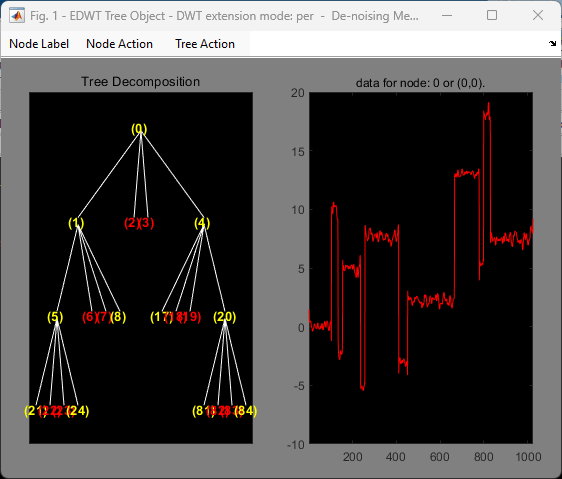## Build Wavelet Tree Objects

The following sections explain how to extend the toolbox with new objects through four examples.

### Building a Wavelet Tree Object (WTREE)

This example creates a new class of objects: WTREE.

Starting from the class DTREE and overloading the methods `split` and `merge`, we define a wavelet tree class.

To plot a WTREE, the DTREE `plot` method is used.

The definition of the new class is described below.

Class WTREE (parent class: DTREE)

#### Fields

 `dtree` Parent object `dwtMode` DWT extension mode `wavInfo` Structure (wavelet information)

#### wavInfo Structure information

 `wavName` Wavelet Name `Lo_D` Low Decomposition filter `Hi_D` High Decomposition filter `Lo_R` Low Reconstruction filter `Hi_R` High Reconstruction filter

#### Methods

 `wtree` Constructor for the class WTREE. `merge` Merge (recompose) the data of a node. `split` Split (decompose) the data of a terminal node.

### Working With Wavelet Tree Objects (WTREE)

1-D Object

```load noisbloc x = noisbloc;```

Define the level and the wavelet.

```lev = 3; wav = 'db2';```

Create the wavelet tree.

`t = wtree(x,lev,wav);`

Plot the wavelet tree. The approximations are labeled in yellow and the details are labeled in red. The detail nodes cannot be split.

`plot(t)`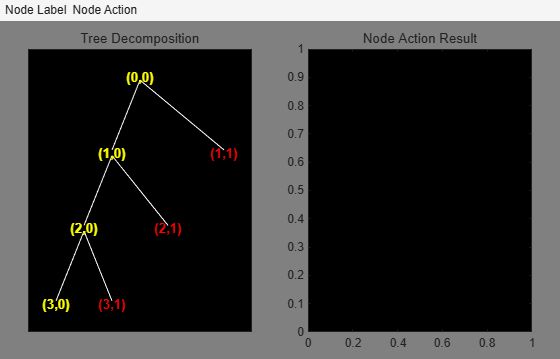Change Node Label from Visualize to Split-Merge, and then click node (7), to get this figure: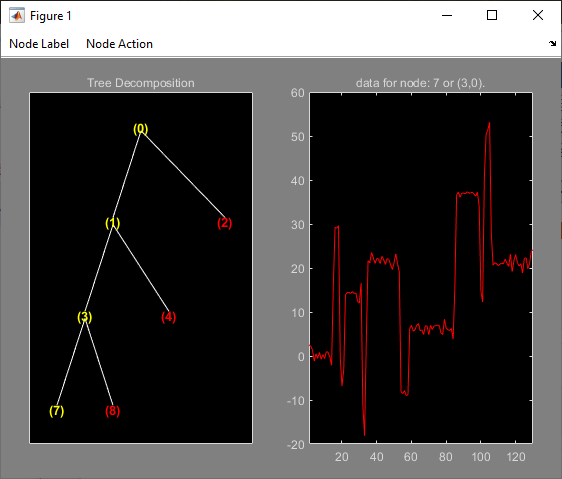Change Node Action from Visualize to Split-Merge, and merge node (3). Then change Node Action from Split-Merge to Visualize, and click node (3) to get this figure: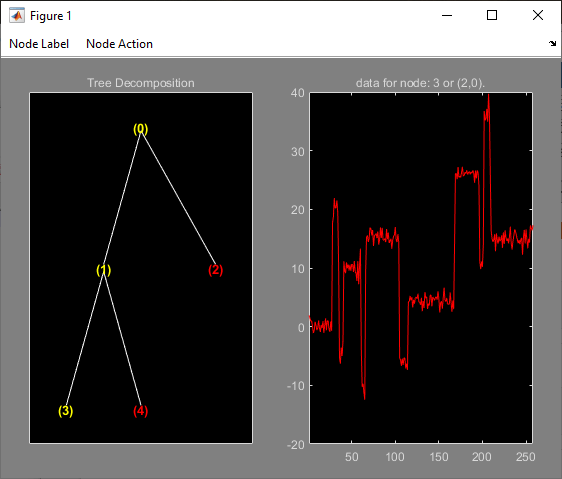2-D Object

`load woman`

Define the level and the wavelet.

```lev = 2; wav = 'db2';```

Create the wavelet tree.

`t = wtree(X,lev,wav);`

Plot the tree.

`plot(t)`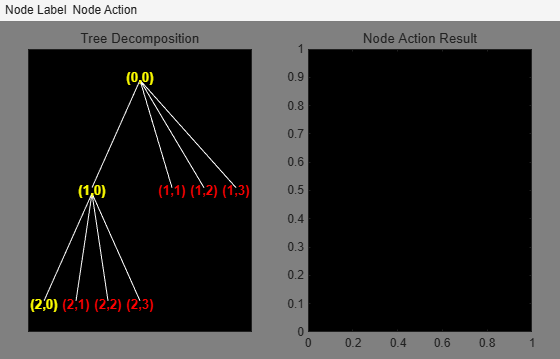Change Node Label from Depth_Position to Index. Click the node (5). You get the following plot.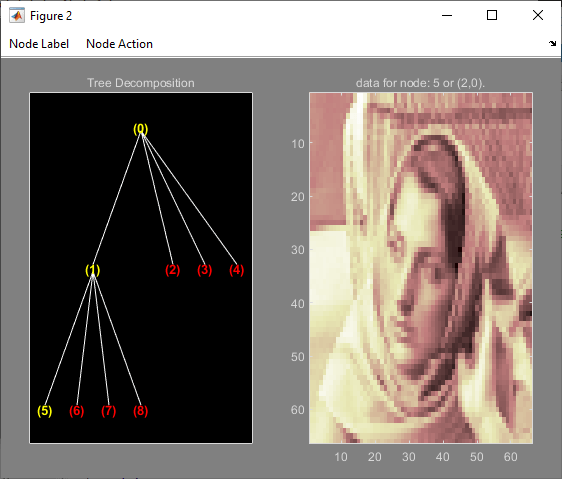Click the node (2). You obtain the following plot.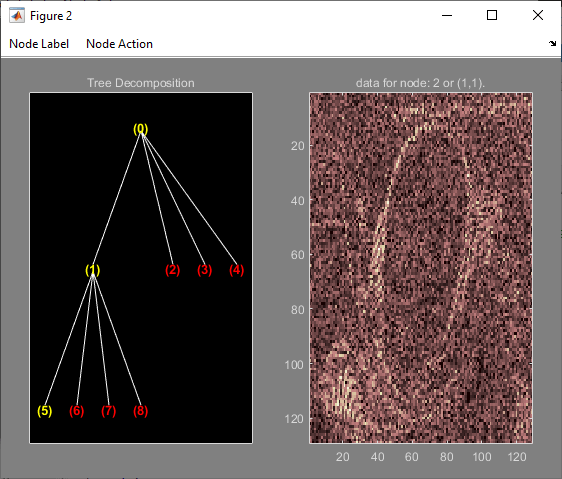Change Node Action from Visualize to Split-Merge. Split the node (5). Change Node Action from Split-Merge to Visualize. Click the node (21). You obtain the following plot.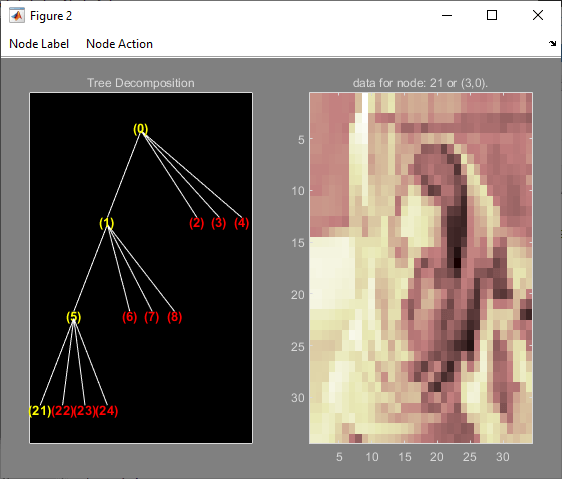### Building a Right Wavelet Tree Object (RWVTREE)

This example creates a new class of objects: RWVTREE.

We define a right wavelet tree class starting from the class WTREE and overloading the methods `split`, `merge`, and `plot` (inherited from DTREE).

The `plot` method shows how to add Node Labels.

The definition of the new class is described below.

Class RWVTREE (parent class: WTREE)

#### Fields

 `dummy` Not used `wtree` Parent object

#### Methods

 `rwvtree` Constructor for the class RWVTREE. `merge` Merge (recompose) the data of a node. `plot` Plot RWVTREE object. `split` Split (decompose) the data of a terminal node.

### Working With Right Wavelet Tree Objects (RWVTREE)

1-D Object

```load noisbloc x = noisbloc;```

Define the level and the wavelet.

```lev = 3; wav = 'db2';```

Create the wavelet tree.

`t = rwvtree(x,lev,wav);`

Plot the tree. The approximations are labeled in yellow and the details are labeled in red. The detail nodes cannot be split.

`plot(t)`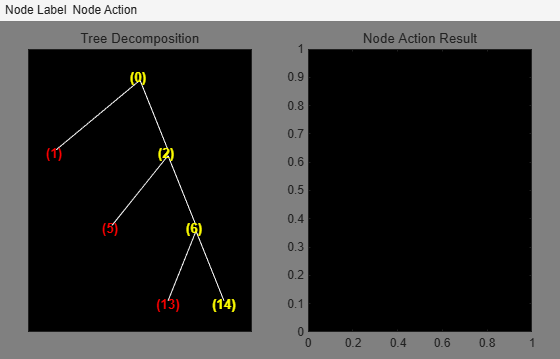Change Node Action from Visualize to Split-Merge. Merge the node (6). Change Node Action from Split-Merge to Visualize. Click the node (6). You obtain the following plot.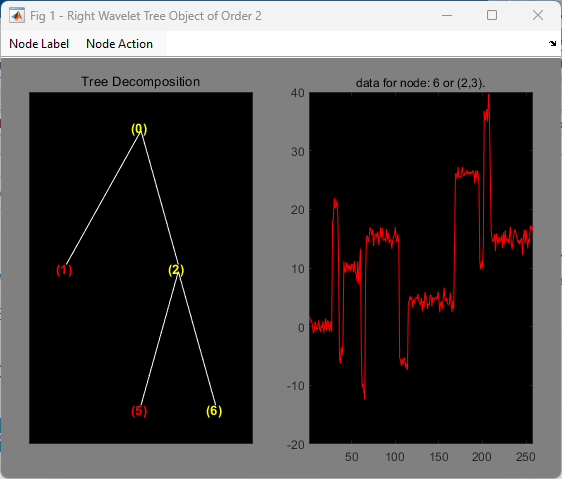2-D Object

`load woman`

Define the level and the wavelet.

```lev = 2; wav = 'db2';```

Create the wavelet tree.

`t = wtree(X,lev,wav);`

Plot the tree.

`plot(t)`Click the node (2,0). You get the following plot.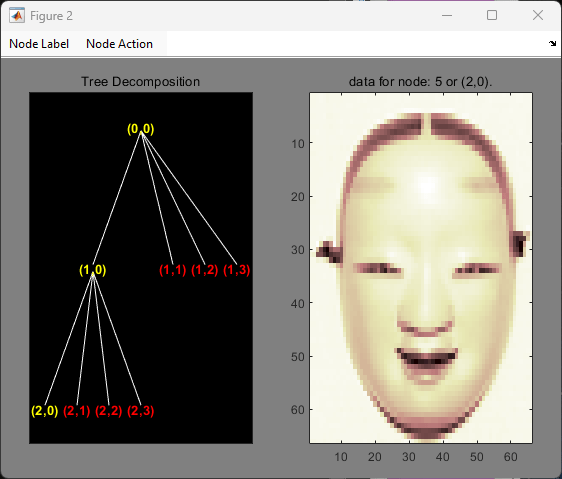Change Node Action from Visualize to Split-Merge. Split the node (2,0). Change Node Action from Split-Merge to Visualize. Click the node (3,0). You obtain the following plot.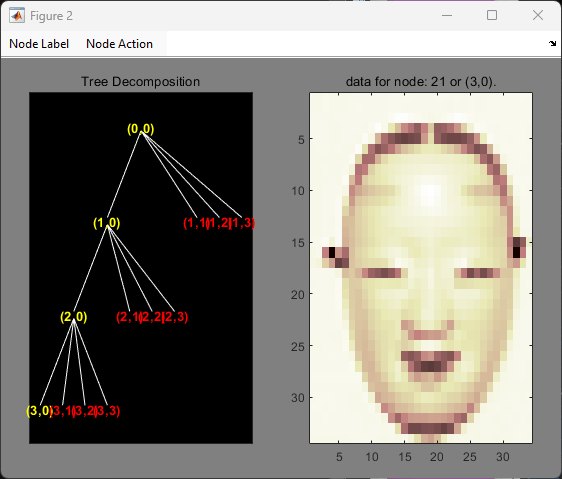### Building a Wavelet Tree Object (WVTREE)

This example creates a new class of objects: WVTREE.

We define a wavelet tree class starting from the class WTREE and overloading the methods `get`, `plot`, and `recons` (all inherited from DTREE).

The `split` and `merge` methods of the class WTREE are used.

The `plot` method shows how to add Node Labels and Node Actions.

The definition of the new class is described below.

Class WVTREE (parent class: WTREE)

#### Fields

 `dummy` Not used `wtree` Parent object

#### Methods

 `wvtree` Constructor for the class WVTREE. `get` Get WVTREE object field contents. `plot` Plot WVTREE object. `recons` Reconstruct node coefficients.

### Working With Wavelet Tree Objects (WVTREE)

1-D Object

``` load noisbloc x = noisbloc;```

Define the level and the wavelet.

```lev = 3; wav = 'db2';```

Create the wavelet tree.

`t = wvtree(x,lev,wav);`

Plot the tree. The approximations are labeled in yellow and the details are labeled in red. The detail nodes cannot be split.

` plot(t)`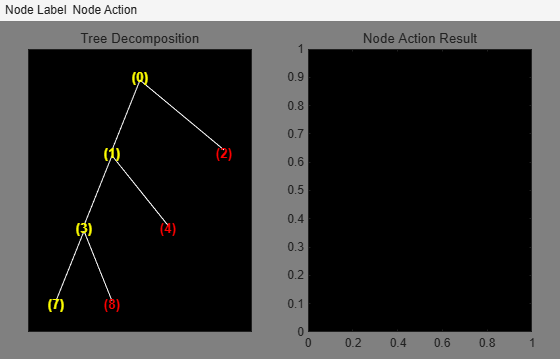Change Node Action from Visualize to Split-Merge. Merge the node (3). Change Node Action from Split-Merge to Reconstruct. Click the node (3). You obtain the following plot.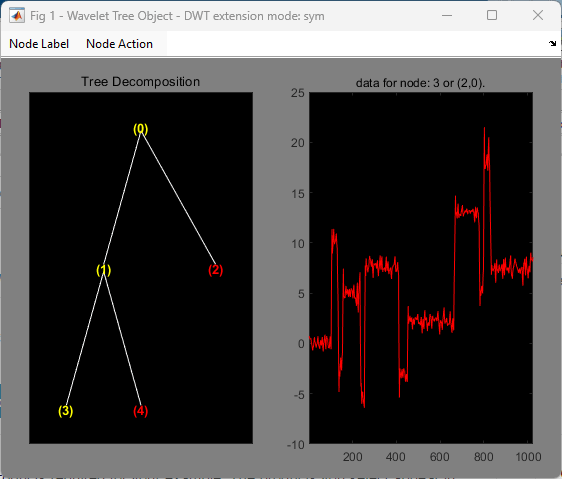2-D Object

`load woman`

Define the level and the wavelet.

```lev = 2; wav = 'db1';```

Create the wavelet tree.

`t = wvtree(X,lev,wav);`

Plot the tree.

`plot(t)`Click the node (5). You get the following plot.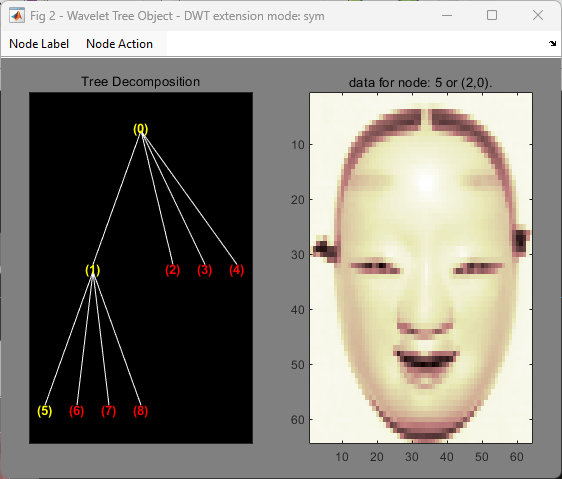Click the node (2). You obtain the following plot.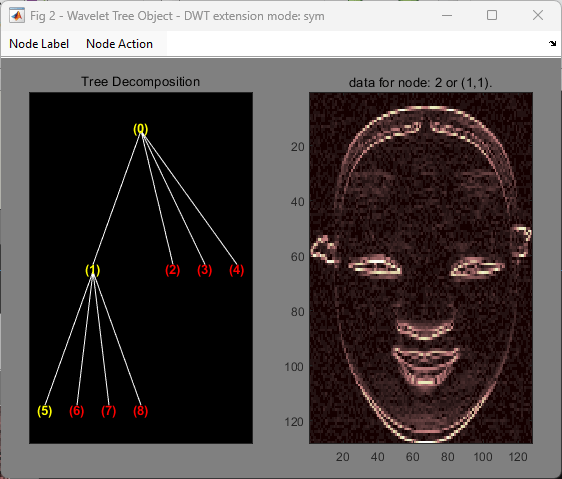Change Node Action from Visualize to Split-Merge. Split the node (5). Change Node Action from Split-Merge to Reconstruct. Click the node (21). You obtain the following plot.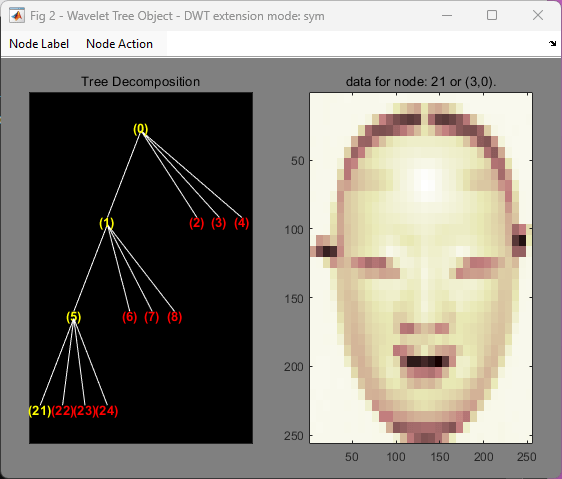### Building a Wavelet Tree Object (EDWTTREE)

This example creates a new class of objects: EDWTTREE.

We define an ε-DWT tree class starting from the class DTREE and overloading the methods `merge`, `plot`, `recons`, and `split`.

For more information on the ε-DWT, see the section ε-Decimated DWT.

The `plot` method shows how to add Node Labels, Node Actions, and Tree Actions.

The definition of the new class is described below.

Class EDWTTREE (parent class: DTREE)

#### Fields

 `dtree` Parent object `dwtMode` DWT extension mode `wavInfo` Structure (wavelet information)

#### Fields Description

`wavInfo`

 `wavName` Wavelet Name `Lo_D` Low Decomposition filter `Hi_D` High Decomposition filter `Lo_R` Low Reconstruction filter `Hi_R` High Reconstruction filter

#### Methods

 `edwttree` Constructor for the class EDWTTREE. `merge` Merge (recompose) the data of a node. `plot ` Plot EDWTTREE object. `recons ` Reconstruct node coefficients. `split` Split (decompose) the data of a terminal node.

### Working With Wavelet Tree Object (EDWTTREE)

```load noisbloc x = noisbloc;```

Define the level and the wavelet.

```lev = 2; wav = 'haar';```

Create the wavelet tree.

`t = edwttree(x,lev,wav);`

Plot the tree. The approximations are labeled in yellow and the details are labeled in red. The detail nodes cannot be split. The title of the figure contains the DWT extension mode used (`'per'` in the present example) and the name of the denoising method.

`plot(t)`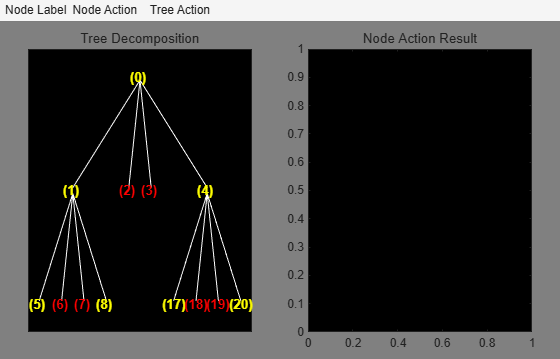Click the node (0). You obtain the following plot.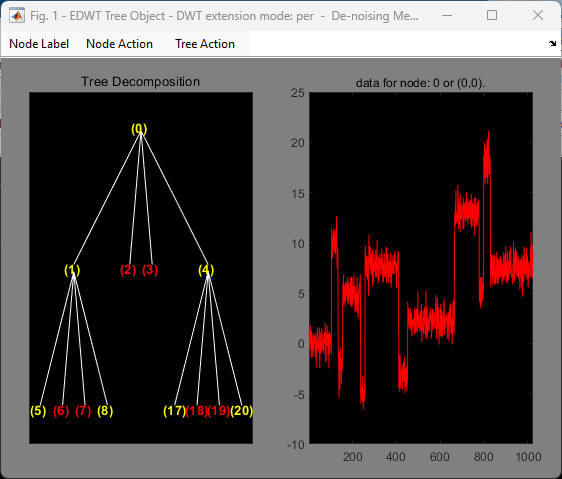Change Node Action from Visualize to Split-Merge. Split the nodes (5) and (20).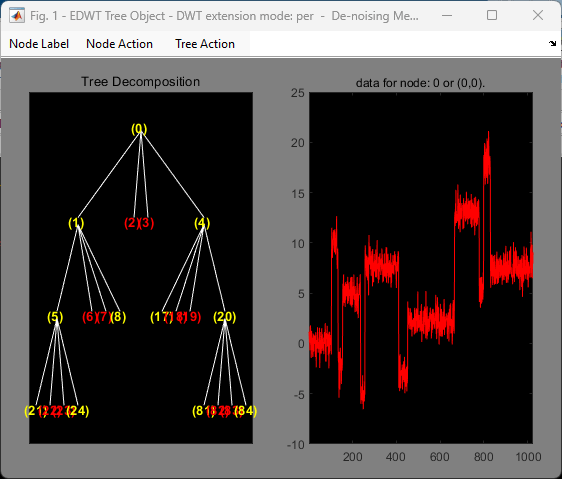Select Tree Action: De-noise. Click the node (0). You obtain the following plot.